Mobile QR Code1. (School of Electronic and Electrical Engineering, Kyungpook National University, Daegu 702-201, Korea)
2. (Korea Multi-purpose Accelerator Complex, Korea Atomic Energy Research Institute, Gyeongju 38180, Korea)
3. (Power Semiconductor Research Center, Korea Electrotechnology Research Institute, Changwon 51543, Korea )

Gallium nitride (GaN), 3D architecture, field-effect transistor (FET), vertical transistor, power transistor

## I. INTRODUCTION

The demand for wide bandgap semiconductors has dramatically increased in application fields such as electric vehicles, the military, and national defense. Therefore, the development of next-generation power devices based on wide bandgap materials has become an important issue (1,2). Gallium-nitride (GaN) has the high critical electric field and the high electron mobility due to the two-dimensional electron gas (2-DEG) in the heterostructure of AlGaN/GaN, and it is suitable for power electronics (3,4). However, GaN devices with the lateral channel are not suitable for extremely high-power devices. Because the lateral GaN devices require a long gate-drain distance to achieve high threshold voltage (Vth) and high breakdown voltage (VB), it results in the increase of device area. Otherwise, the vertical device has the advantage of high V$_{\mathrm{B}}$ and wide drift current region without increasing the chip size (5-8). Therefore, vertical GaN devices could be promising candidate device for high-power electronic applications. For general vertical devices, the cylindrical device is adopted for its excellent gate controllability (9,10). However, for vertical GaN devices, the performance is highly dependent on the orientation of the sidewall plane. GaN has a wurtzite crystal structure and has various sidewall planes such as m-plane (1-100), a-plane (11-20), and c-plane (0001) (11). Many studies have reported that the m-plane outperforms the a-plane (12,13). However, a direct comparison between a cylindrical device with mixed planes and a hexagonal device with a single crystal plane has not yet been reported for GaN-based vertical trench MOSFETs.

In this study, we present the electrical characteristics of GaN-based vertical trench MOSFETs based on cylindrical and hexagonal structures. Through the 3-D simulations, the electrical performances of GaN-based vertical trench MOSFETs are extracted and evaluated in terms of the current density, V$_{\mathrm{th}}$, and on-resistance (R$_{\mathrm{on}}$) for both cylindrical and hexagonal devices.

Fig. 1. 3-D and cross-sectional schematic diagrams of the hexagonal and the cylindrical structure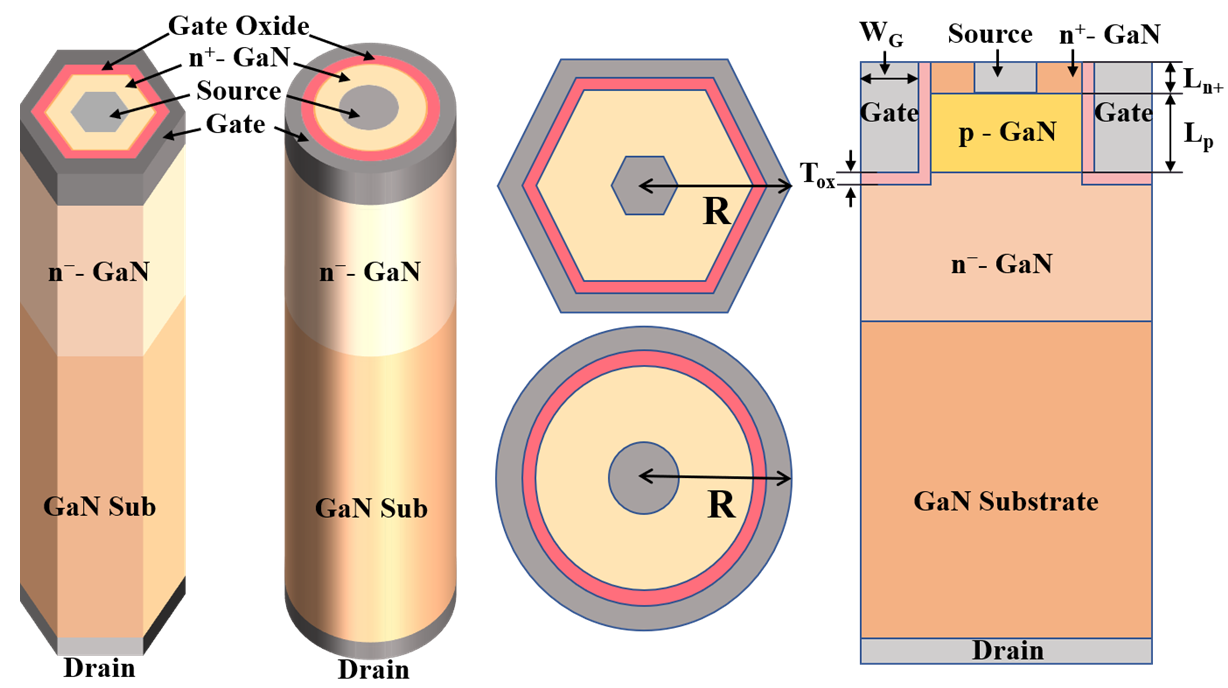Table 1.Parameters for vertical GaN trench MOSFET

 Parameter Value Gate Width (WG) 1 μm Gate Oxide (Al2O3) Thickness (Tox) 80 nm n+-GaN Length (Ln+) 200 nm p+-GaN Length (Lp) 700 nm n- -GaN Length 13 μm n+-GaN Doping Concentration 1 × 1018 cm-3 p+-GaN Doping Concentration 2 × 1018 cm-3 n- -GaN Doping Concentration 9 × 1015 cm-3 n+-GaN Substrate Doping Concentration 6 × 1018 cm-3 Hexagonal Cross-sectional Area (AH) 137.32 μm2 Cylindrical Cross-sectional Area (AC) 166.04 μm2 Radius (R) 7.27 μm

## II. DEVICE STRUCTURE AND SIMULATION

Fig. 1 shows the schematic diagrams of the hexagonal and cylindrical cell structures of GaN-based vertical trench MOSFETs (14). The geometric parameters are summarized in Table 1. The epitaxial structure including the GaN substrate consists of a 13 μm-thick n$^{{-}}$-GaN drift layer, a 700 nm-thick p-GaN channel layer, and a 200 nm-thick n$^{+}$-GaN layer. The n-type doping concentration of n$^{+}$-GaN and n$^{{-}}$-GaN are 6 ${\times}$ 10$^{18}$ cm$^{{-}3}$ and 9 ${\times}$ 10$^{15}$ cm$^{{-}3}$, respectively. The p-type doping concentration of p-GaN is 2 ${\times}$ 10$^{18}$ cm$^{{-}3}$. 80 nm-thick Al$_{2}$O$_{3}$ is used for the gate dielectric (15). This simulation assumes that all acceptors in the p-GaN layer are ionized. In addition, a channel is formed in the p-GaN layer to operate as a conventional enhancement mode transistor. The radius of both devices is equal to 7.27 μm to compare the electric field at the same corner.

Fig. 2. Interface trap density and trap energy level of Al2O3/GaN for m-plane and mixed-plane.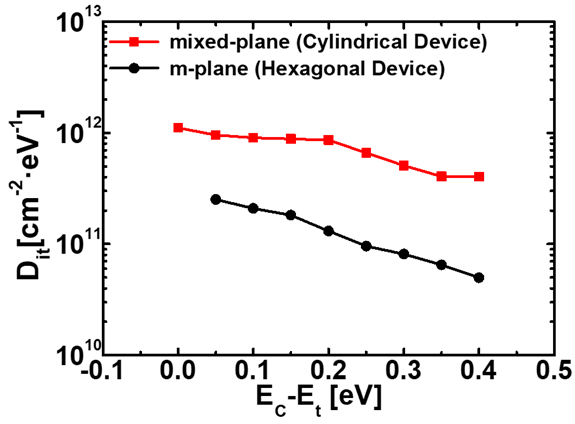Fig. 3. Linear and logarithmic transfer Id-Vg characteristics for the hexagonal and the cylindrical devices at Vd = 0.5 V: (a) without interface trap, (b) with interface trap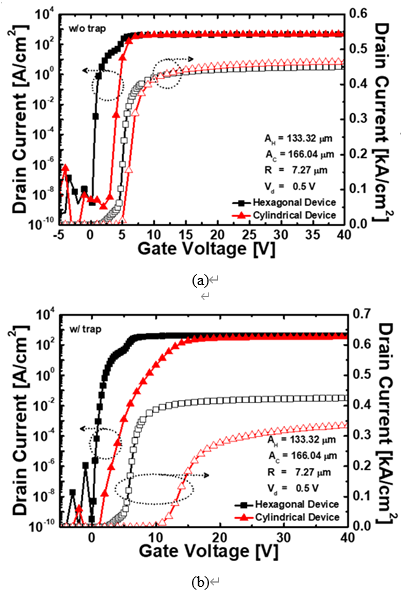The c-plane of GaN is not suitable for the normally-off operation because spontaneous polarization occurs as a polar plane. Generally, the a-plane or m-plane is used as a non- polar plane when fabricating the vertical GaN devices because they can achieve high V$_{\mathrm{th}}$ (16,17). Fig. 2 shows the Al-$_{2}$O$_{3}$/GaN interface trap densities for the m-plane and the mixed-plane (18). The hexagonal device consists of six m-plane sidewalls, and the cylindrical device consists of sidewalls with a mixed-plane of m-plane and a-plane, rather than a single crystal plane. The mixed-plane has higher interface traps than the m-plane because the a-plane has a higher trap density than the m-plane (13). These simulations include various physical models such as the Fermi-Dirac model, the Shockley-Read-Hall recombination model, the low field mobility model, the high field mobility model, and the shock ionization model, which were applied to increase simulation’s accuracy.

Fig. 4. Simulation profiles of the hexagonal and the cylindrical devices at Vg = 40 V showing electric field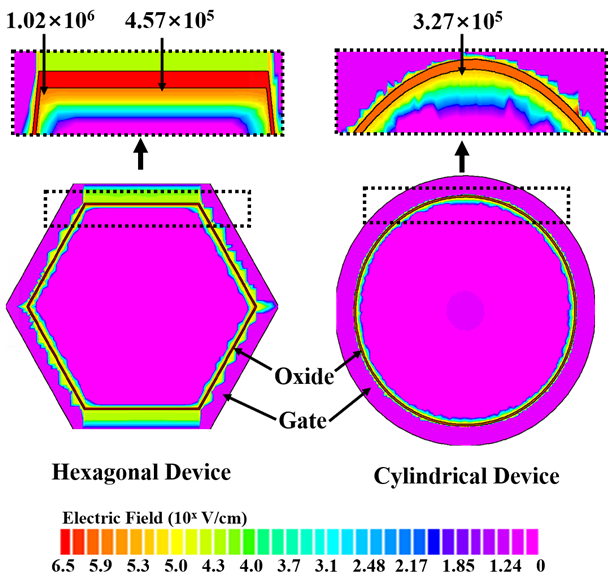## III. RESULT AND DISCUSSION

Fig. 3 shows the drain current (I$_{\mathrm{d}}$)-gate voltage (V$_{\mathrm{g}}$) characteristics for the hexagonal and the cylindrical GaN-based trench MOSFETs at drain voltage (V$_{\mathrm{d}}$) = 0.5 V. The I$_{\mathrm{d}}$ is normalized by the cross-sectional area of the device. Fig. 3(a) is the I$_{\mathrm{d}}$-V$_{\mathrm{g}}$ curve for devices without the interface trap. The V$_{\mathrm{th}}$ for the hexagonal and the cylindrical devices in this case are 4.37 V and 4.99 V, and the I$_{\mathrm{d}}$ are 0.450 kA/cm$^{2}$, and 0.464 kA/cm$^{2}$ at V$_{\mathrm{g }}$= 40 V. The hexagonal device has the smaller perimeter and cross-sectional area than the cylindrical device, so the channel forms faster and has a lower V$_{\mathrm{th}}$. In addition, the hexagonal device such as angled device, the V$_{\mathrm{th}}$ is lower because the electric field is focused on the corner due to the corner effect, and channel forms quickly at the corner (19). The cylindrical device has higher I$_{\mathrm{d}}$ than the hexagonal device due to excellent gate controllability. Fig. 3(b) is the I$_{\mathrm{d}}$-V$_{\mathrm{g}}$ curve for devices with the interface trap. The V$_{\mathrm{th}}$ of the hexagonal and the cylindrical devices in this case are 5.12 V and 11.15 V, and the I$_{\mathrm{d}}$ are 0.425 kA/cm$^{2}$ and 0.335 kA/cm$^{2}$ at V$_{\mathrm{g}}$ = 40 V. Due to the influence of the interface traps on both devices, the V$_{\mathrm{th}}$ is increased and the I$_{\mathrm{d}}$ is decreased. The cylindrical device is more affected by interface traps than the hexagonal device, due to the inclusion of an a-plane with high interface traps. Therefore, despite the excellent gate controllability of the cylindrical device, the electrical performance is inferior to the hexagonal device.

Fig. 5. (a) Logarithmic transfer Id-Vg and transconductance gm-Vg characteristics for the hexagonal device, (b) simulation profiles of cross-section showing electron concentrationFig. 4 shows the electric fields of the hexagonal and the cylindrical devices at V$_{\mathrm{g}}$ = 40 V. The cylindrical device has a smooth perimeter, which leads to a uniform electric field along the surface. On the other hand, the hexagonal device, the electric field is concentrated at the corners due to the sharp corners, as a result having a non-uniform electric field along the surface. Specifically, the electric field of the hexagonal device is 1.02 MV/cm at the corner and 0.46 MV/cm at the edge. Although the electric field of the cylindrical device is slightly different, the result is almost uniform at 0.33 MV/cm.

Fig. 5(a) shows the logarithmic I$_{\mathrm{d}}$-V$_{\mathrm{g}}$ and transconductance (g$_{\mathrm{m}}$)-V$_{\mathrm{g}}$ characteristics of the hexagonal device. Fig. 5(b) shows the cross-sectional electron concentration at each point. As shown in Fig. 5(a), the hump shape occurs in the I$_{\mathrm{d}}$-V$_{\mathrm{g}}$ curve of the hexagonal device. The inversion layer is not formed and the current does not flow well when V$_{\mathrm{g}}$ is 0 V, as shown in Fig. 5(b). The inversion layer begins to be formed at the corner when V$_{\mathrm{g}}$ increases until just before the formation of the hump. When additional V$_{\mathrm{g}}$ is applied and the hump shape occurs, a sufficient inversion layer is formed on the edges as well as the corners, allowing more current to flow. Finally, at V$_{\mathrm{g}}$ = 40 V, the inversion layer of high electron concentration is formed at the edges and corners. This is due to the corner effect shown in Fig. 4, which is the reason the channel is formed rapidly at the corners of the hexagonal device, resulting in lower V$_{\mathrm{th}}$ and a difference in the formation of an inversion layer between edges and corners, resulting in the hump shape occurs.

Fig. 6 shows the I$_{\mathrm{d}}$-V$_{\mathrm{d}}$ characteristic of the hexagonal and the cylindrical devices. The R$_{\mathrm{on}}$ is calculated in the linear region of V$_{\mathrm{d}}$ = 0.5 V and V$_{\mathrm{g}}$ = 40 V. Fig. 6(a) for devices without the interface trap shows that the cylindrical device has a higher current density than the hexagonal device. Also, the R$_{\mathrm{on}}$ is 1.16 mΩ·cm$^{2}$ of the cylindrical device, which is lower than 1.20 mΩ·cm$^{2}$ of the hexagonal device. However, Fig. 6(b) for devices with the interface trap shows that the hexagonal device has a higher current density than the cylindrical device. This means that the hexagonal device has higher channel mobility and lower R$_{\mathrm{on}}$ than the cylindrical device. The calculated R$_{\mathrm{on}}$ is 1.24 mΩ·cm$^{2}$ of the hexagonal device and 1.66 mΩ·cm$^{2}$ of the cylindrical device. This is because the a-plane included in the mixed-plane of the cylindrical device has higher trap density and lower interface quality than the m-plane. These results indicate that the GaN-based vertical trench MOSFET is highly dependent on the orientation of the channel sidewall plane.

Fig. 7 shows the off-state I$_{\mathrm{d}}$-V$_{\mathrm{d}}$ characteristics of the hexagonal and the cylindrical devices when V$_{\mathrm{g}}$ is 0 V. The V$_{\mathrm{B}}$ of the hexagonal and the cylindrical devices are 2,660 V and 2,682 V, respectively. The V$_{\mathrm{B}}$ of the hexagonal device is slightly lower than that of the cylindrical device because the electric field is focused on the corners. The hexagonal device has not only better electrical performances than the cylindrical device but also the V$_{\mathrm{B}}$ of only about 1\% lower than the cylindrical device.

Fig. 6. Output Id-Vd characteristics for the hexagonal and the cylindrical devices at Vg = 40 V: (a) without interface trap, (b) with interface trapThe V$_{\mathrm{th}}$, I$_{\mathrm{d,}}$ and R$_{\mathrm{on}}$ of the analyzed hexagonal and cylindrical devices are summarized in Table 2. As shown in Table 2, the cylindrical device shows superior electric performances when there are no interface traps. However, considering the interface traps along the crystal plane orientation, the electrical performances of both devices are degraded. Specifically, the cylindrical device shows inferior electrical performances as compared to the hexagonal device due to many interface traps.

Fig. 7. Off-state Id-Vd Characteristic for the hexagonal and the cylindrical devices at Vg = 0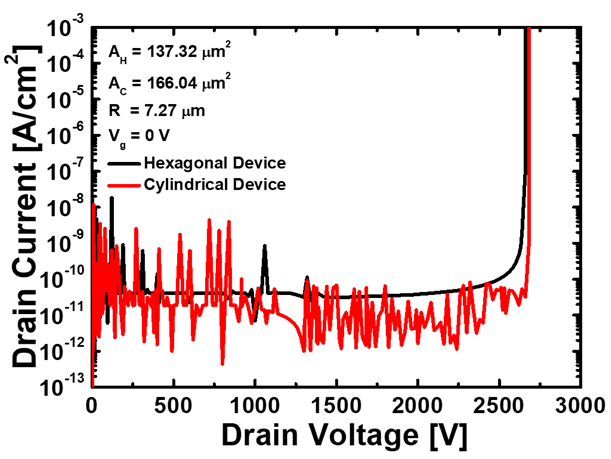Table 2. Parameters Metrics of the analyzed devices

 Electrical Performance Metrics Hexagonal device Cylindrical device w/o trap w/ trap w/o trap w/ trap Vth [V] 4.37 5.12 4.99 11.15 Id [kA/cm2] 0.450 0.425 0.464 0.335 Ron [mΩ·cm2] 1.20 1.24 1.16 1.66

## IV. CONCLUSIONS

This study analyzed the effect of crystal orientations for the sidewall plane on the electrical performances of GaN-based vertical trench MOSFETs with hexagonal and cylindrical structures. The GaN-based vertical trench MOSFET’s electrical performances are highly dependent on the orientation of the sidewall plane of the channel. Electrical performances decreased in both devices due to the influence of the interface traps. However, it is shown that the hexagonal device consists of m-plane and has fewer interface traps than the cylindrical device consists of mixed-plane, it has better electrical performances despite the negative effects due to the corner effect. Therefore, to enhance the performances of the device, it is advantageous to design the crystal orientation toward the m-plane. The results of this study provide insight into the electrical properties of GaN-based vertical trench MOSFETs, as well as design guidelines.

### ACKNOWLEDGMENTS

This work was supported by the National Research Foundation of Korea (NRF) grant funded by the Korea government (MSIT) (No. NRF-2020R1A2C1005087). This study was supported by the BK21 FOUR project funded by the Ministry of Education, Korea (4199990113966), by the Ministry of Trade, Industry \& Energy (MOTIE) (10080513) and Korea Semiconductor Research Consortium (KSRC) support program for developing the future semiconductor devices. This research was supported by Basic Science Research Program through the National Research Foundation of Korea (NRF) funded by the Ministry of Education (NRF-2021R1A6A3A13039927). This research was supported by National R\&D Program through the National Research Foundation of Korea (NRF) funded by Ministry of Science and ICT (2021M3F3A2A03017764). The EDA tool was supported by the IC Design Education Center (IDEC), Korea.

### REFERENCES

1
Jang Won-Ho, Dec 2020, P-GaN Gated AlGaN/GaN E-mode HFET Fabricated with Selective GaN Etching Process., JOURNAL OF SEMICONDUCTOR TECHNOLOGY AND SCIENCE, pp. 485-4902
Ouduangvilai Konepachith, Dec 2019, Study of Gate Leakage Current on AlGaN/GaN MOSHEMTs with Atomic Layer Deposited Al2O3 Gate Oxide, JOURNAL OF SEMICONDUCTOR TECHNOLOGY AND SCIENCE, pp. 540-5503
Choton , Jahid Chowdhury , Feb 2019, Design and Characterization of 2DEG Structure of a Gallium Nitride HEMT., IEEE 2019 International Conference on Robotics, Electrical and Signal Processing Techniques (ICREST)., pp. 486-4894
Syaranamual , Sep 2016, Role of two-dimensional electron gas (2DEG) in AlGaN/GaN high electron mobility transistor (HEMT) ON-state degradation., Microelectronics Reliability, Vol. 64, No. 21, pp. 589-5935
Kanechika Masakazu, May 2007, A vertical insulated gate AlGaN/GaN heterojunction field-effect transistor., Japanese Journal of Applied Physics, Vol. 46, pp. 503-5056
Srabanti Chowdhury, L. Brian, Jun 2008 , Enhancement and depletion mode AlGaN/GaN CAVET with Mg-ion-implanted GaN as current blocking layer., IEEE Electron Device Letters, Vol. 29, No. 6, pp. 543-5457
Min Sun, Apr 2017, High-performance GaN vertical fin power transistors on bulk GaN substrates., IEEE Electron Device Letters, Vol. 38, No. 4, pp. 509-5128
Tohru Oka, Jan 2014, Vertical GaN-based trench metal oxide semiconductor field-effect transistors on a free-standing GaN substrate with blocking voltage of 1.6 kV., Applied Physics Express p. 021002, Vol. 7, No. 29
H. Takato, Dec 1988, High performance CMOS surrounding gate transistor (SGT) for ultra high density LSIs., IEEE Technical Digest., International Electron Devices Meeting, pp. 11-1410
Kim Sung Yoon, May 2015 Aug 2016, Electrical characteristics of enhancement-mode n-channel vertical GaN MOSFETs and the effects of sidewall slope., Journal of Electrical Engineering and Technology, Vol. 10, pp. 1131-113711
T. Wang, Topical Review: Development of overgrown semi-polar GaN for high efficiency green/yellow emission., Semiconductor Science and Technology, p. 093003, Vol. 31, No. 912
Chirag Gupta, Nov 2016, Comparing electrical performance of GaN trench-gate MOSFETs with a-plane and m-plane sidewall channels., Applied Physics Express p. 121001, Vol. 9, No. 1213
Ye Jia, Jun 2017, Interface characterization of atomic layer deposited high-k on non-polar GaN., Journal of Applied Physics p. 154104, Vol. 122, No. 1514
Tohru Oka, Apr 2015, 1.8 mΩ· cm2 vertical GaN-based trench metal–oxide–semiconductor field-effect transistors on a free-standing GaN substrate for 1.2-kV-class operation., Applied Physics Express p. 054101, Vol. 8, No. 515
Chang Y. C., Jun 2008, Inversion-channel GaN metal-oxide-semiconductor field-effect transistor with atomic-layer-deposited Al2O3 as gate dielectric., Applied Physics Letters p. 053504, Vol. 93, No. 516
Houqiang Fu, Oct 2018, Nonpolar vertical GaN-on-GaN p–n diodes grown on free-standing m-plane GaN substrates., Applied Physics Express, Vol. 11, No. 1117
Tetsuya Fujiwara, Jan 2009, Enhancement-mode m-plane AlGaN/GaN heterojunction field-effect transistors., Applied Physics Express, p. 011001, Vol. 2, No. 118
Terirama Thingujam, Jul 2021, A simulation study on the effects of interface charges and geometry on vertical GAA GaN nanowire MOSFET for low-power application., IEEE Access, pp. 101447-101453, Vol. 919
Luca De Michielis, Sep 2010, Corner effect and local volume inversion in SiNW FETs., IEEE transactions on nanotechnology, Vol. 10, No. 4, pp. 810-816## Author

Geon Uk Kim received a B.Sc. degree in electronic engineering from the School of Electronics Engi-neering (SEE), Kyungpook National University (KNU), Daegu, South Korea, in 2021, where he is currently pursuing the M.Sc. degree in School of Electronic and Electrical Engineering, Kyungpook National University (KNU), Daegu, South Korea.

His research interests include the design, fabrication, and characterization of GaN devices and capacitor-less 1T-DRAM transistors.

Young Jun Yoon received the B.S. and Ph.D. degrees in electronics engineering from Kyungpook National University, Daegu, Korea, in 2013 and 2019, respectively.

He is currently postdoctoral researcher with Korea Multi-purpose Accelera-tor Complex, Korea Atomic Energy Research Institute (KAERI).

His research interests include design, fabrication, and characterization of logic transistor and memory.

Jae Hwa Seo received the B.S. and Ph.D. degree in Electronics Engi-neering from the School of Electronics Engineering, Kyungpook National University (KNU), Daegu, Korea, in 2012, 2018.

He worked as a Post Doc. in electrical engineering from School of Electrical Engineering and Computer Science (EECS), Seoul National University (SNU), Seoul, Korea, in 2018 to 2019.

Now, he has worked as reseacher at Power Semiconductor Research Center, Korea Electrotechnology Research Institute.

His research interests include the design, fabrication and characterization of V-NAND/1T-DRAM devices, nano-scale CMOS, tunneling FETs, and compound/silicon based transistors.

Min Su Cho received a B.Sc. degree in computer engineering from the College of Electrical and Computer Engineering, Chungbuk National University (CBNU), Cheongju, South Korea, in 2015, and an M.Sc. degree from the School of Electronics Engineering (SEE), Kyungpook National University (KNU), and Ph.D. degree in Electronics Engineering from the School of Electronic and Electrical Engineering.

He is currently postdoctoral researcher with KNU.

His research interests include the design, fabrication, and characterization of compound CMOS, tunneling FETs, and III–V compound transistors.

Sang Ho Lee received the B.Sc. degree in electronics engineering from the School of Electronics Engineering (SEE), Kyungpook National University (KNU), Daegu, South Korea, in 2019, where he is currently pursuing the Ph.D. in School of Electronic and Electrical Engineering, Kyungpook National University (KNU), Daegu, South Korea.

His research interests include the design, fabrication, and characterization of gate-all-around logic devices and capacitor-less 1T-DRAM transistors.

Jin Park received a B.Sc. degree in electronic engineering from the School of Electronics Engineering (SEE), Kyungpook National University (KNU), Daegu, South Korea, in 2020, where she is currently pursuing the M.Sc. degree in School of Electronic and Electrical Engineering, Kyungpook National University (KNU), Daegu, South Korea.

Her research interests include the design, fabrication, and characterization of gate-all-around logic devices and capacitor-less 1T-DRAM transistors.

Hee Dae An received the B.Sc. degree in School of Electronic Engineering, Kumoh National Institute of Techology (KIT), Gumi, South Korea, in 2019, where he is currently pursuing the M.Sc. degree in School of Electronic and Electrical Engineering, Kyungpook National University (KNU), Daegu, South Korea.

His research interests include the design, fabrication, and characterization of capacitor-less 1T-DRAM transistors and vertical GaN power devices.

So Ra Min received the B.Sc. degree in Electronic Engineering from the School of Electronics Engineering, Yeungnam University (YU), Gyeong-san, North Gyeongsang, South Korea, in 2020, where she is currently pursuing the M.Sc. degree in school of Electronic and Electrical Engineering.

Her research interests include the design, fabrication, and characterization of GaN devices and capacitor-less 1T-DRAM transistors.

In Man Kang received the B.S. degree in electronic and electrical engineering from School of Electronics and Electrical Engi-neering, Kyungpook National University (KNU), Daegu, Korea, in 2001, and the Ph.D. degree in electrical engineering from School of Electrical Engineering and Computer Science (EECS), Seoul National University (SNU), Seoul, Korea, in 2007.

He worked as a teaching assistant for semiconductor process education from 2001 to 2006 at Inter-university Semiconductor Research Center (ISRC) in SNU.

From 2007 to 2010, he worked as a senior engineer at Design Technology Team of Samsung Electronics Company.

In 2010, he joined KNU as a full-time lecturer of the School of Electronics Engineering (SEE).

Now, he is currently working as a professor.

His current research interests include CMOS RF modeling, silicon nanowire devices, tunneling transistor, low-power nano CMOS, and III-V compound semiconductors. He is a member of IEEE EDS.